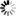# The University of Dodoma Library

Normal view MARC view ISBD view

# Essential mathematical methods for the physical sciences / K.F. Riley, M.P. Hobson.

Material type:TextPublisher: Cambridge ; New York : Cambridge University Press, 2011Description: xvi, 829 p. : ill. ; 26 cm. + Student solution manual.ISBN: 9780521761147 (hardback); 9780521141024 (pbk. : student solution manual).DDC classification: 510 Other classification: SCI040000
Contents:
Machine generated contents note: 1. Matrices and vector spaces; 2. Vector calculus; 3. Line, surface and volume integrals; 4. Fourier series; 5. Integral transforms; 6. Higher-order ODEs; 7. Series solutions of ODEs; 8. Eigenfunction methods; 9. Special functions; 10. Partial differential equations; 11. Solution methods for PDEs; 12. Calculus of variations; 13. Integral equations; 14. Complex variables; 15. Applications of complex variables; 16. Probability; 17. Statistics; Appendices; Index.
Summary: "The mathematical methods that physical scientists need for solving substantial problems in their fields of study are set out clearly and simply in this tutorial-style textbook. Students will develop problem-solving skills through hundreds of worked examples, self-test questions and homework problems. Each chapter concludes with a summary of the main procedures and results and all assumed prior knowledge is summarized in one of the appendices. Over 300 worked examples show how to use the techniques and around 100 self-test questions in the footnotes act as checkpoints to build student confidence. Nearly 400 end-of-chapter problems combine ideas from the chapter to reinforce the concepts. Hints and outline answers to the odd-numbered problems are given at the end of each chapter, with fully-worked solutions to these problems given in the accompanying Student Solutions Manual. Fully-worked solutions to all problems, password-protected for instructors, are available at www.cambridge.org/essential"--
Tags from this library: No tags from this library for this title.Average rating: 0.0 (0 votes)
Item type Current location Call number Copy number Status Date due BarcodeBooks Library, College of Natural and Mathematical Sciences (CNMS)
Special Reserve
QA37.3 .R55 2011 (Browse shelf) 1-1 Available 1828261

Includes index.

Machine generated contents note: 1. Matrices and vector spaces; 2. Vector calculus; 3. Line, surface and volume integrals; 4. Fourier series; 5. Integral transforms; 6. Higher-order ODEs; 7. Series solutions of ODEs; 8. Eigenfunction methods; 9. Special functions; 10. Partial differential equations; 11. Solution methods for PDEs; 12. Calculus of variations; 13. Integral equations; 14. Complex variables; 15. Applications of complex variables; 16. Probability; 17. Statistics; Appendices; Index.

"The mathematical methods that physical scientists need for solving substantial problems in their fields of study are set out clearly and simply in this tutorial-style textbook. Students will develop problem-solving skills through hundreds of worked examples, self-test questions and homework problems. Each chapter concludes with a summary of the main procedures and results and all assumed prior knowledge is summarized in one of the appendices. Over 300 worked examples show how to use the techniques and around 100 self-test questions in the footnotes act as checkpoints to build student confidence. Nearly 400 end-of-chapter problems combine ideas from the chapter to reinforce the concepts. Hints and outline answers to the odd-numbered problems are given at the end of each chapter, with fully-worked solutions to these problems given in the accompanying Student Solutions Manual. Fully-worked solutions to all problems, password-protected for instructors, are available at www.cambridge.org/essential"--

There are no comments for this item.